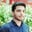Related Tags

python
pandas

# How to use DataFrame.lt() in pandasSalman Yousaf

## What is the function DataFrame.lt()?

The wrapper function DataFrame.lt() compares two DataFrames. It checks whether or not one DataFrame is less than the other.

DataFrame.lt() is equivalent to < in Python, but has an additional support to select axis, ('columns' or 'index'), and level for index comparison. The axis decides the direction of the operation either column-wise or index-wise. The level helps match indexes on the passedpasses in arguments MultiIndex level.

### Syntax

DataFrame.lt(other, axis='columns', level=None)

### Parameters

• other: This can either be a series, sequence, or DataFrame. It can be a single or a multiple values data structure.
• axis=0 or axis='index': This means we calculate along with the index. axis=1 or axis='columns': This means we calculate along with columns.
• level=None: This helps broadcast single indexed or multi-indexed values.

### Return value

It returns a boolean DataFrame. A boolean DataFrame contains boolean values.

### Code

Here's an example of the implementation of the DataFrame.lt() method:

# load pandas library
import pandas as pd
# creating first dataframe
df1 = pd.DataFrame({'X': [25, 120, 120, 10],
'Y': [25, 350, 200, 35]},
index=['A', 'B', 'C', 'D'])
# creating second dataframe
df2 = pd.DataFrame({'X': [150, 90, 350, 120, 130, 330],
'Y': [160, 450, 30, 560, 235, 356]},
index=[['R1', 'R1', 'R1', 'R2', 'R2', 'R2'],
['A', 'B', 'C', 'A', 'B', 'C']])
# invoking df.le() to calculate less than between
print(df1.lt(df2, level=1))

### Explanation

• Lines 1–4: We create a first DataFrame named df1. It contains two variables and four observations.
• Line 8: We create a second DataFrame as df2. It contains two variables with six observations.
• Line 13: df1.lt(df2, level=1) calculates the "less than" operation between df1 and df2. Here, level=1 means that calculation will be performed along with the columns. It will print the boolean DataFrame on the console.

RELATED TAGS

python
pandas

CONTRIBUTORSalman Yousaf
RELATED COURSES

View all Courses

Keep Exploring

Learn in-demand tech skills in half the time